# Play with Stream in Java8 and get to know Stream from scratch

Posted Jun 28, 202010 min read

Author:litesky

I believe that everyone has heard of Java8 Stream, but you may not use it or you are not familiar with it. The article will take everyone to use it from scratch, step by step, and take you to the peak of Stream.

### Operator

What is an operator? The operator is a kind of processing work on the data, a processing program; just like the factory workers perform a processing program on the products on the assembly line.

Stream operators are roughly divided into two types:intermediate operators and termination operators

### Intermediate operator

For the data flow, after the intermediate operator executes the specified processing procedure, the data flow can still be passed to the next level of operator.

There are 8 kinds of intermediate operators(excluding parallel and sequential, these two operations do not involve processing operations on the data stream):

map(mapToInt, mapToLong, mapToDouble) conversion operator, such as A->B, here provides default conversion int, long, double operator.
flatmap(flatmapToInt,flatmapToLong,flatmapToDouble) Flattening operations such as flattening int[]{2,3,4} into 2, 3, 4 means changing from one original data to 3 data, here default Provides operators for flattening into int, long, and double.
Limit current limit operation, for example, there are 10 in the data stream, I can use it as long as the first three.
distint deduplication operation, deduplication of repeated elements, the bottom uses the equals method.
filter filter operation to filter unwanted data.
peek picks out operations, if you want to perform certain operations on the data, such as:read, edit, etc.
skip Skip operation, skip some elements.
sorted(unordered) Sorting operation, sorting elements, provided that the Comparable interface is implemented, of course, you can also customize the comparator.

### Termination operator

After the intermediate processing operation, it is the turn of the termination operator; the termination operator is used to collect or consume the data. The data will not flow down to the termination operation. The termination operator can only be used once.

The collect operation collects all data. This operation is very important. The officially provided Collectors provide many collectors. It can be said that the core of Stream lies in Collectors.
The count operation counts the final number of data.
findFirst, findAny search operation, find the first, find any one The returned type is Optional.
noneMatch, allMatch, anyMatch match operation, whether there are elements that meet the conditions in the data stream The return value is a bool value.
The min and max operations require a custom comparator to return the maximum and minimum values in the data stream.
The reduce reduction operation reduces the value of the entire data stream to one value. The bottom of count, min, and max is to use reduce.
forEach, forEachOrdered traversal operations, here is the consumption of the final data.
toArray Array operation, convert the elements of the data stream into an array.
Only Stream is introduced here, and IntStream, LongStream, and DoubleStream are not involved. These three streams implement some unique operators, which I will introduce in subsequent articles. Reply to "Interview Question Aggregation" in the public account of Java Friend, and send you a collection of interview summary for major companies.

Having said so much, it is not enough to just introduce these operators; as the saying goes, practice produces real knowledge. So, Let s go.

### Code Walkthrough

Stream's series of operations must use termination operations, otherwise the entire data stream will not flow, that is, processing operations will not be performed.

map, you can see that the map operator requires input of a Function function is an interface instance, the function is to convert T type to R type.The map operation converts the original word to the length of each single, using the String's own length() method, which returns an int. Here I use the lambda expression directly, please let the reader know about the lambda expression.

``````public class Main {

public static void main(String[]args) {
Stream.of("apple","banana","orange","waltermaleon","grape")
.map(e->e.length()) //The length of the word converted to int
.forEach(e->System.out.println(e)); //output
}
}``````

Of course, this is also possible. Here, member function references are used. In order to facilitate readers' understanding, lambda expressions will be used instead of function references in subsequent examples.

``````public class Main {

public static void main(String[]args) {
Stream.of("apple","banana","orange","waltermaleon","grape")
.map(String::length) //The length of the word converted to int
.forEach(System.out::println);
}
}``````

The result is shown in the figure:mapToInt converts the elements in the data stream to Int, which defines the type of conversion Int, the resulting stream is IntStream, and the result can only be converted to int.``````public class Main {

public static void main(String[]args) {
Stream.of("apple", "banana", "orange", "waltermaleon", "grape")
.mapToInt(e -> e.length()) //Convert to int
.forEach(e -> System.out.println(e));
}
}``````

mapToInt as shown: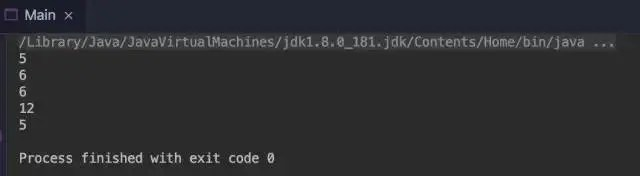mapToLong, mapToDouble and mapToInt are similar

``````public class Main {

public static void main(String[]args) {
Stream.of("apple", "banana", "orange", "waltermaleon", "grape")
.mapToLong(e -> e.length()) //Convert to long, essentially int but there is automatic type conversion
.forEach(e -> System.out.println(e));
}
}``````

mapToLong as shown:``````public class Main {

public static void main(String[]args) {
Stream.of("apple", "banana", "orange", "waltermaleon", "grape")
.mapToDouble(e -> e.length()) //Convert to Double, automatic type conversion to Double
.forEach(e -> System.out.println(e));
}
}``````

mapToDouble as shown:The function of flatmap is to flatten the elements, flatten the flattened elements into a Stream, and merge these Streams into a Stream in series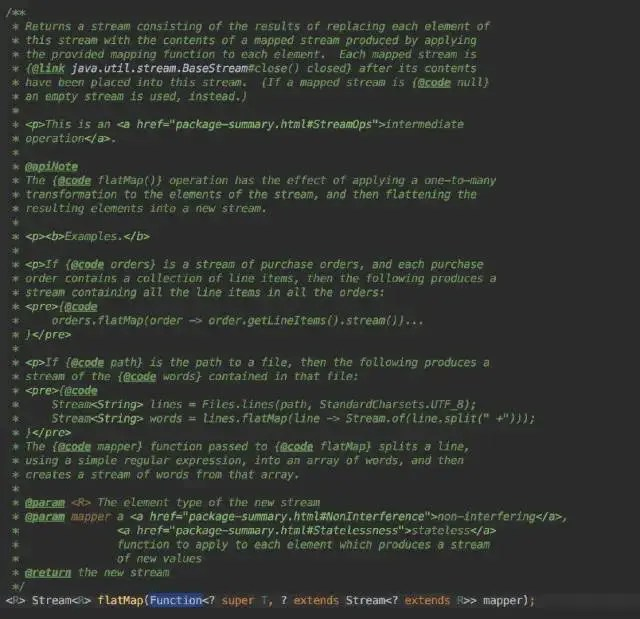``````public class Main {

public static void main(String[]args) {
Stream.of("a-b-c-d","e-f-i-g-h")
.flatMap(e->Stream.of(e.split("-")))
.forEach(e->System.out.println(e));

}
}``````

flatmap as shown: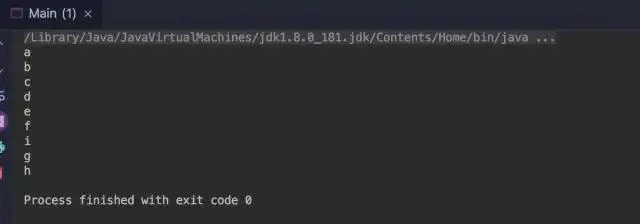flatmapToInt, flatmapToLong, flatmapToDouble are similar to flatMap, except that the type is limited, so here are no examples.

limit limit the number of elements, only need to pass in long type to indicate the maximum number of limits

``````public class Main {

public static void main(String[]args) {
Stream.of(1,2,3,4,5,6)
.limit(3) //limit three
.forEach(e->System.out.println(e)); //The first three 1, 2, 3 will be output
}
}``````

limit as shown: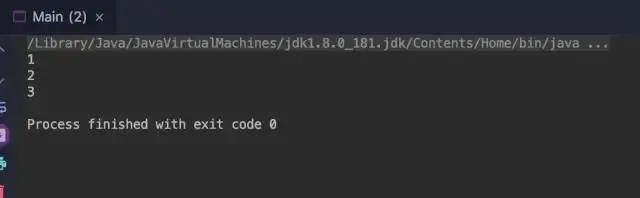``````public class Main {

public static void main(String[]args) {

Stream.of(1,2,3,1,2,5,6,7,8,0,0,1,2,3,1)
.distinct() //Deduplication
.forEach(e->System.out.println(e));

}
}``````

distinct as shown: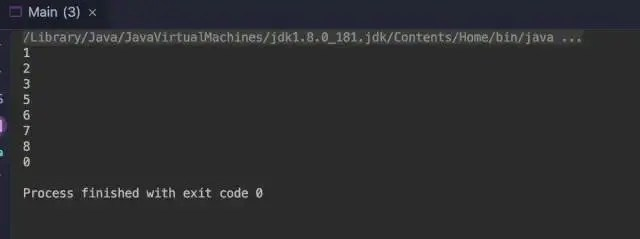filter to filter certain elements, those that do not meet the filtering conditions will not be able to enter the downstream of the stream

``````public class Main {

public static void main(String[]args) {
Stream.of(1,2,3,1,2,5,6,7,8,0,0,1,2,3,1)
.filter(e->e>=5) //Filter less than 5
.forEach(e->System.out.println(e));
}
}``````

filter as shown: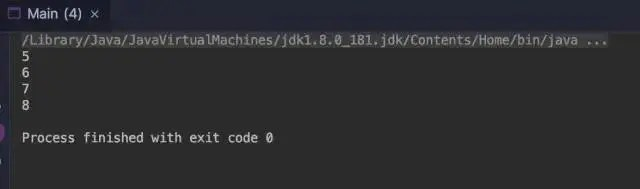peek picking, picking out elements, which can be understood as early consumption

``````public class Main {

public static void main(String[]args) {

User w = new User("w",10);
User x = new User("x",11);
User y = new User("y",12);

Stream.of(w,x,y)
.peek(e->{e.setName(e.getAge()+e.getName());}) //Reset name becomes age+name
.forEach(e->System.out.println(e.toString()));

}

static class User {

private String name;

private int age;

public User(String name, int age) {
this.name = name;
this.age = age;
}

public String getName() {
return name;
}

public void setName(String name) {
this.name = name;
}

public int getAge() {
return age;
}

public void setAge(int age) {
this.age = age;
}

@Override
public String toString() {
return "User{" +
"name='" + name +'\'' +
", age=" + age +
'}';
}
}

}``````

Peek as shown:skip skip element

``````public class Main {

public static void main(String[]args) {
Stream.of(1,2,3,4,5,6,7,8,9)
.skip(4) //skip the first four
.forEach(e->System.out.println(e)); //The output result should only be 5, 6, 7, 8, 9
}
}``````

skip as shown: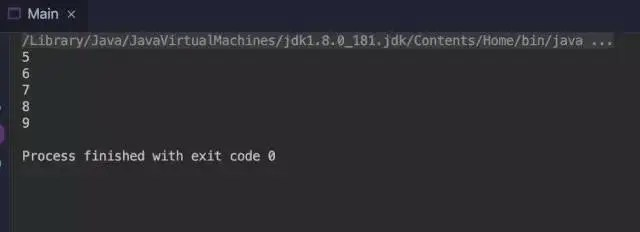Sorted sorting The bottom layer depends on Comparable implementation, and can also provide custom comparator
Here Integer implements the comparator

``````public class Main {

public static void main(String[]args) {
Stream.of(2,1,3,6,4,9,6,8,0)
.sorted()
.forEach(e->System.out.println(e));
}
}``````

The sorted default comparator is shown in the figure: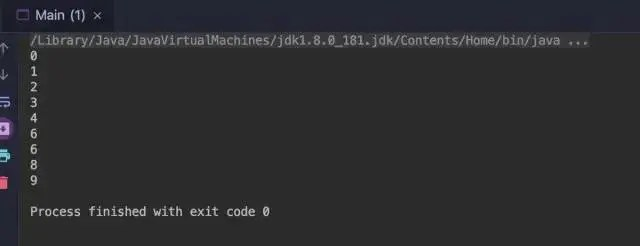Custom comparison is used here, of course, User can implement the Comparable interface

``````public class Main {

public static void main(String[]args) {

User x = new User("x",11);
User y = new User("y",12);
User w = new User("w",10);

Stream.of(w,x,y)
.sorted((e1,e2)->e1.age>e2.age?1:e1.age==e2.age?0:-1)
.forEach(e->System.out.println(e.toString()));

}

static class User {

private String name;

private int age;

public User(String name, int age) {
this.name = name;
this.age = age;
}

public String getName() {
return name;
}

public void setName(String name) {
this.name = name;
}

public int getAge() {
return age;
}

public void setAge(int age) {
this.age = age;
}

@Override
public String toString() {
return "User{" +
"name='" + name +'\'' +
", age=" + age +
'}';
}
}

}``````

As shown: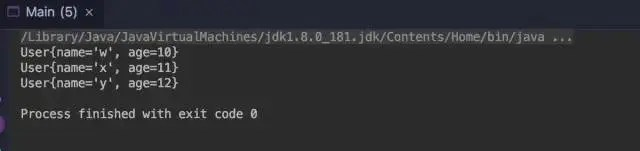collect collection, using the collector provided by the system can collect the final data stream into List, Set, Map and other containers.
Here I use collect to collect the elements into a set

``````public class Main {

public static void main(String[]args) {
Stream.of("apple", "banana", "orange", "waltermaleon", "grape")
.collect(Collectors.toSet()) //set container
.forEach(e -> System.out.println(e));
}
}``````

Huh? , Doesn't it mean that the termination operator can only be used once, why is forEach called here? forEach is not only an operator in Stream or a syntactic sugar in various collections, I don't believe it, let's try it. Reply to "Interview Question Aggregation" in the public account of Java Friend, and send you a collection of interview summary for major companies.

``````public class Main {

public static void main(String[]args) {

Set<String> stringSet = Stream.of("apple", "banana", "orange", "waltermaleon", "grape")
.collect(Collectors.toSet()); //The result of the collection is set
stringSet.forEach(e->System.out.println(e)); set syntactic sugar forEach
}``````

The result is shown in the figure: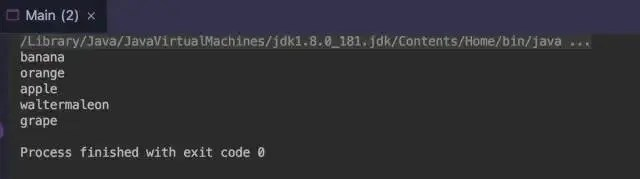count counts the number of elements in the data stream and returns a long type

``````public class Main {

public static void main(String[]args) {

long count = Stream.of("apple", "banana", "orange", "waltermaleon", "grape")
.count();

System.out.println(count);
}
}``````

count as shown: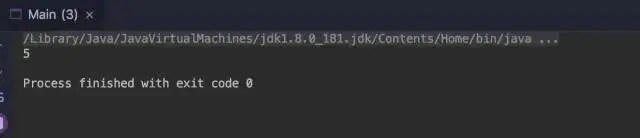findFirst gets the first element in the stream
Find the first element here

``````public class FindFirst {

public static void main(String[]args) {
Optional<String> stringOptional = Stream.of("apple", "banana", "orange", "waltermaleon", "grape")
.findFirst();
stringOptional.ifPresent(e->System.out.println(e));
}
}``````

The result of findFirst is shown in the figure:findAny Get any element in the stream

``````public class FindAny {

public static void main(String[]args) {
Optional<String> stringOptional = Stream.of("apple", "banana", "orange", "waltermaleon", "grape")
.parallel()
.findAny(); //The result of each return under the parallel stream may be the same or different
stringOptional.ifPresent(e->System.out.println(e));
}
}``````

FindAny uses the results under parallel streaming:

Output orange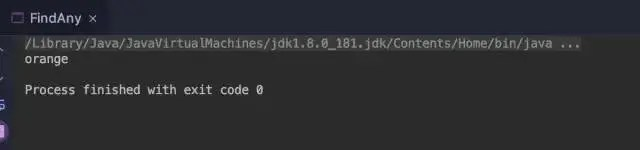Exported banananoneMatch data stream that no element matches the condition
The role here is to determine that none of the data stream has elements equal to aa, but there is aa in the stream, so the final result should be false

``````public class NoneMatch {

public static void main(String[]args) {
boolean result = Stream.of("aa","bb","cc","aa")
.noneMatch(e->e.equals("aa"));
System.out.println(result);
}
}``````

noneMatch as shown:AllMatch and anyMatch are all matches, and one is any match. It is similar to noneMatch, so we will not give examples here.

min The smallest one, passed into the comparator, may not be(if the data stream is empty)

``````public class Main {

public static void main(String[]args) {

Optional<Integer> integerOptional = Stream.of(0,9,8,4,5,6,-1)
.min((e1,e2)->e1.compareTo(e2));

integerOptional.ifPresent(e->System.out.println(e));

}``````

Min as shown: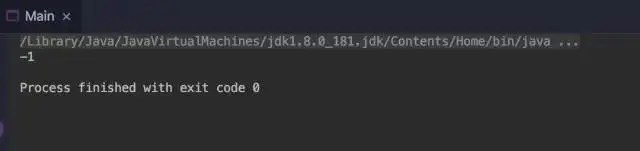The largest of the max elements needs to be passed to the comparator, or may not be(when the stream is Empty)

``````public class Main {

public static void main(String[]args) {

Optional<Integer> integerOptional = Stream.of(0,9,8,4,5,6,-1)
.max((e1,e2)->e1.compareTo(e2));

integerOptional.ifPresent(e->System.out.println(e));

}
}``````

Max as shown:Reduce is a reduction operation, all elements are reduced to one, such as summing, multiplying, etc. all elements.
Here an addition is implemented, specifying the initial value

``````public class Main {
public static void main(String[]args) {

int sum = Stream.of(0,9,8,4,5,6,-1)
.reduce(0,(e1,e2)->e1+e2);
System.out.println(sum);
}
}``````

reduce as shown:forEach
forEach has actually been seen before, iterating over each data iteration

forEachOrdered is suitable for iteration in the case of parallel streams, which can guarantee the order of iteration
Here the numbers are output in parallel

``````public class ForEachOrdered {
public static void main(String[]args) {
Stream.of(0,2,6,5,4,9,8,-1)
.parallel()
.forEachOrdered(e->{
}
}``````

forEachOrdered as shown: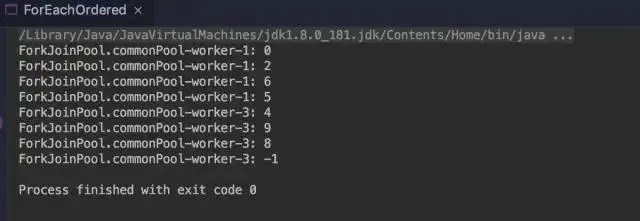toArray into an array, you can provide a custom array generator

``````public class ToArray {
public static void main(String[]args) {
Object[]objects=Stream.of(0,2,6,5,4,9,8,-1)
.toArray();

for(int i = 0; i <objects.length; i++) {
System.out.println(objects[i]);
}
}
}``````

toArray as shown: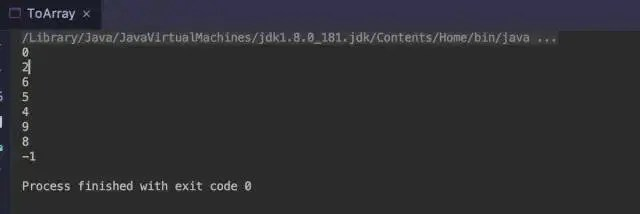to sum up

Java8 Stream brings everyone to realize here, if you can follow my article to knock through each example, I believe you can master the initial usage of these operators.

WeChat search:Java small coffee show responded to the "manual" to obtain a Java core interview manual + a linux practical command manual.